## ORIGINAL RESEARCH article

Front. Water, 16 June 2022
Sec. Water and Hydrocomplexity
https://doi.org/10.3389/frwa.2022.886964

# Estimating Lake Water Volume With Regression and Machine Learning MethodsChelsea Delaney1,Xiang Li1,Kerry Holmberg1,Bruce Wilson1,Adam Heathcote2 andJohn Nieber1*
• 1Department of Bioproducts and Biosystems Engineering, University of Minnesota, St. Paul, MN, United States
• 2St. Croix Watershed Research Station, Science Museum of Minnesota, Marine on St. Croix, MN, United States

The volume of a lake is a crucial component in understanding environmental and hydrologic processes. The State of Minnesota (USA) has tens of thousands of lakes, but only a small fraction has readily available bathymetric information. In this paper we develop and test methods for predicting water volume in the lake-rich region of Central Minnesota. We used three different published regression models for predicting lake volume using available data. The first model utilized lake surface area as the sole independent variable. The second model utilized lake surface area but also included an additional independent variable, the average change in land surface area in a designated buffer area surrounding a lake. The third model also utilized lake surface area but assumed the land surface to be a self-affine surface, thus allowing the surface area-lake volume relationship to be governed by a scale defined by the Hurst coefficient. These models all utilized bathymetric data available for 816 lakes across the region of study. The models explained over 80% of the variation in lake volumes. The sum difference between the total predicted lake volume and known volumes were <2%. We applied these models to predicting lake volumes using available independent variables for over 40,000 lakes within the study region. The total lake volumes for the methods ranged from 1,180,000- and 1,200,000-hectare meters. We also investigated machine learning models for estimating the individual lake volumes and found they achieved comparable and slightly better predictive performance than from the three regression analysis methods. A 15-year time series of satellite data for the study region was used to develop a time series of lake surface areas and those were used, with the first regression model, to calculate individual lake volumes and temporal variation in the total lake volume of the study region. The time series of lake volumes quantified the effect on water volume of a dry period that occurred from 2011 to 2012. These models are important both for estimating lake volume, but also provide critical information for scaling up different ecosystem processes that are sensitive to lake bathymetry.

## Introduction

Fresh water is a crucial resource to humans. With an ever-changing environment, we need to be better prepared to protect it. One of the most important freshwater bodies are lakes. While the surface area of all lakes covers <4% of the global landmass and the total volume of water is a small fraction of total terrestrial freshwater, they are home to a wide range of biodiverse ecosystems (McDonald et al., 2012). The ecosystem functioning of lakes provides tangible ecologic and economic value, yet key information such as lake datasets that contain basic morphological and hydrologic characteristics needed to determine these functions are missing (Hollister et al., 2011; Crétaux et al., 2016). Lake volume and maximum lake depth are vital components in many lake functions related to the physical, biological, and chemical processes within a lake. For example, the volume of a lake can affect the water residence time which in turn can affect the nutrient dynamics and primary productivity (Sobek et al., 2011) as well as the zooplankton dynamics of a lake (Obertegger et al., 2007). With missing or inaccurate data, the prediction of these functions is not as precise as they could be, making it more difficult to quantify the changes that may occur within these environments (Sobek et al., 2011; Crétaux et al., 2016; Messager et al., 2016).

As two important parameters determining the nature of circulation processes and biogeochemical processes in lakes, data on lake volume and lake depth are scarce. Even the available data in many parts of the world are merely present for only a very small fraction of the total number of lakes. For instance, in Minnesota, ‘the land of lakes,' the number of lakes with detailed bathymetric data is <2% of the total number of lakes in the state. Given that current technology makes it impractical to directly measure bathymetric information at large scales, it becomes necessary to develop predictive models for these parameters using the information that is available. At present, a widely used approach is to estimate lake volume with lake surface area data. Models for lake volume using lake surface area were among the first models developed and include the work by Håkanson and Karlsson (1984). Improvements in lake volume models were made by including a second prediction variable that involved some measure of the land surface topography in the area surrounding a lake. The idea of this second variable is that the topography of the surface surrounding a lake would reflect the topography of the lake bottom. Studies that involved a prediction variable representing the surrounding topography include Håkanson and Peters (1995), Hollister et al. (2011), and Sobek et al. (2011).

A modification of the lake buffer topography variable was proposed by Heathcote et al. (2015). In this study, they used the change in surface elevation in a buffer area surrounding the lake, with the buffer area scaled according to lake surface area. Heathcote et al. applied this model to the data for 433 lakes located in different geographic regions in the southern part of the Province of Quebec (Canada). In doing so, the model explained 95% of the variation in lake volume.

While the Heathcote et al. (2015) method predicted lake volumes using self-similar scaling, the Cael et al. (2017) method developed a model assuming that the land surface is self-affine. The scaling of such surfaces has been shown theoretically to be related to the Hurst coefficient. Since lake water fills in the depressions of the land surface, a description of the surface as being self-affine should provide a theoretical background for predicting the volume of water in the depressions. According to the theory of such self-affine surfaces, the volume of the depression will be proportional to the depressional surface area raised to some exponent. This exponent can be shown to be calculated from the Hurst coefficient, which itself can be related to the fractal dimension of the surface. For the earth surface, the Hurst coefficient has been determined to be about 0.4 ± 0.1 for the spatial scale relevant to lakes (see for example Renard et al., 2013).

In their study, Cael et al. (2017) predicted lake volumes on a global scale with vastly different regions and topographic features. The model is meant to be used to predict the total volume and mean depth of a collection of lakes. However, the model can be used to estimate the volume for individual lakes, but these are determined on a statistical basis. Their estimate of the total volume of lakes globally was 199,000 km3, which is lower than previous estimates of 210,000 km3.

Both Heathcote et al. (2015) and Cael et al. (2017) methods estimate lake volume in a statistical regression model. Statistical models are elegant in their solid theoretical foundation, interpretability, and easy implementation. Nevertheless, their ability to handle non-linearity and complex prediction problems are also constrained by their simple model architecture. Recently, machine learning (ML) methods have become a popular approach to model complex non-linearities from scientific data and their contributions to tackle water-related problems have been previously acknowledged (Shen et al., 2018). Despite the wide applicability of machine learning, their use in lake volume prediction, to our knowledge, has not been explored. Thus, we have additionally developed and applied machine learning models to predict lake volume using limited lake bathymetric data and compared this technique to the performance of the regression models.

The ability of ML to solve predictive problems (Sejnowski, 2020) has already made its scientific applications span a diversity of fields. Among them, ML applications in hydrology have also experienced unprecedented progress (Shen et al., 2018). Kratzert et al. (2018, 2019) built machine learning models to predict catchment scale streamflow using weather forcing data and achieves state-of-the-art performance, which also scientifically advances the development in hydrologic regionalization. Jia et al. (2020) coupled physical knowledge into machine learning and builds knowledge-guided machine learning models to model lake temperature. Shukla et al. (2022) applied machine learning methods and Gaussian process modeling techniques to predict discharge with hydrologic knowledge in complex stage-discharge relationships. Additionally, machine learning has also been applied to map lake spreading areas (Deoli et al., 2021) and flooding regions (Avand et al., 2022).

For this paper, we tested the ability of several methods to predict lake volumes in the central region of Minnesota (USA), a region that has over 40,000 lakes (Delaney, 2019). The objective of this study was to predict volumes of lakes to better understand lake processes using readily available, remotely sensed data. The methods included a model using just lake surface area, the model of Heathcote et al. (2015) using lake surface area and near lake topography, the model of Cael et al. (2017) using lake surface area and assuming self-affine surfaces, and methods based on conventional machine learning tools with lake surface area and near lake topography as independent variables. In addition to testing the ability of these models to predict lake volume for one point in time, we also applied the lake surface area regression model to determine the temporal variability in total lake water volume for the entire region for the period 2002–2015.

## Methods

The database used for developing the regression models and the machine learning models was derived from archived lake data available from the Minnesota Department of Natural Resources (Minnesota Department of Natural Resources, 2017). The available data was for 816 lakes, with known volumes and a shapefile of each lake with corresponding bathymetric data. These 816 lakes ranged from volumes of 104 to greater than 107 m3 with known maximum depths, average depths, and surface areas for each lake. A summary of each lake size category is given in Table 1. The developed regression models were then applied to the other lakes in the study region. The hydrography data for these other lakes, absent depth or volumes, were also available from the MnDNR (Department of Natural Resources Division of Fish Wildlife, 2014). The distribution of these lakes, a total of 40,054, is illustrated in Figure 1. The boundaries of the Hydrologic Unit Code (HUC)-8 watersheds, 17 of them, in the region are shown in the illustration. Lake Mille Lacs is noticeable in the northeastern part of the study region by its large size. It contains nearly 25% of the total lake water volume in the 17 HUC-8 watersheds. To better illustrate the range and variability in predictions of lake volume, and because the bathymetric data of large lakes such as Lake Mille Lacs are usually well-defined due to their large economic and recreational value, this lake was excluded from the estimates of total regional lake water volume that follow.

TABLE 1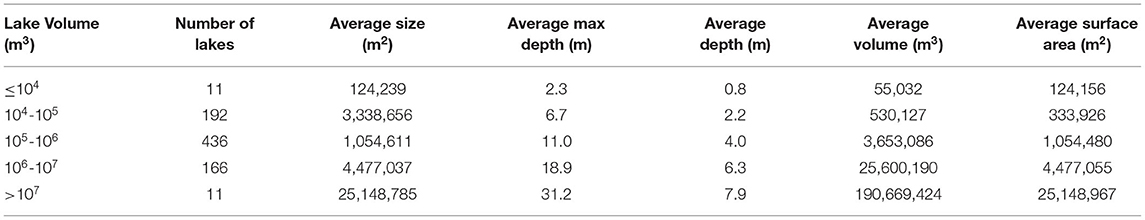Table 1. Averages of morphology traits from 816 surveyed lakes provided by Minnesota Department of Natural Resources (DNR).

FIGURE 1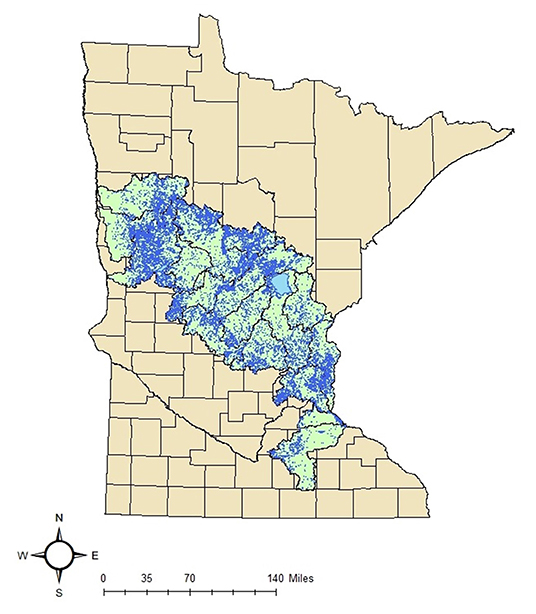Figure 1. Map of 40,054 lakes contained within 17 HUC-8 watersheds of central Minnesota used in lake volume prediction.

Data for the temporal variation of lake surface area was acquired from satellite data provided by the Global Surface Water (GSW) observations program (Pekel et al., 2016). These data were acquired for the period 2002–2015.

### Method Using Surface Area Alone

The first model developed was one using lake surface area only, with the equation being

where a and b are empirical constants. A regression model was developed by regressing log-transformed lake volume on log-transformed lake surface area for the 816 lake dataset.

### Heathcote Method

The original concept of using lake surface area and land surface slope came from Håkanson and Peters (1995) who suggested using an empirical model that calculated lake volume from lake surface area and maximum slope of the catchment from 95 lakes in Sweden. While the lake volume model was able to explain a high percentage of the variability in volume, the model requires catchment area data which may not always be available in some locations. Sobek et al. (2011) improved this concept by using surface area and the maximum slope of the land surface within a static buffer of 50 m around each lake, for a total of 6,130 lakes, to calculate lake volume within Sweden resulting in the lake volume model that explained 92% of the variability in volume. Heathcote et al. (2015) further developed this method to predict lake volume as well as maximum depth, by using the lake surface area and the average change in land surface elevation within a near-lake buffer with the buffer length dependent on the lake surface area. This allowed the lake's buffer area to be proportional to the size of the lake rather than a static buffer distance as done by Sobek et al. (2011).

Heathcote et al. (2015) found the average change in elevation between the surrounding terrestrial landscape and the lake surface to be the best predictor of bathymetric properties, lake volume, and maximum depth (Heathcote et al., 2015). The terrestrial buffer surrounding the lake was used because of the assumption that the elevation change surrounding the lake was formed by the same geomorphic process forming the elevation change within the lake and that the slope of the surrounding topography is near to that of the slope of the lake bottom (Hollister et al., 2011). Due to not being able to calculate the slope occurring under the water because that information is not available, the method uses elevation change surrounding the lake as an independent variable to predict the lake volume. The concept is that by studying the relationship between the morphology of a lake and the surrounding area, lake volumes can be predicted without detailed bathymetric data. Based on their empirical testing, Heathcote et al. (2015) found that the length of buffer should be 25% of the equivalent diameter (D) of the lake surface, where $D=2\sqrt{\frac{A}{\pi }}$ and A is the lake surface area. In our application of the Heathcote et al. (2015) method, the topography for each buffer of Minnesota lakes was calculated using a 1/3 arc-second Digital Elevation map (DEM) (~10 m) (U.S. Geological Survey, 2017).

The prediction equation for lake volume based on the Heathcote et al. (2015) approach is given by

where DE25 corresponds to the average elevation change within the buffer of length equal to 25% of the equivalent lake surface diameter, and c and d are empirical parameters. This regression equation, in log transformed form, log10V = clog10A +dlog10DE25, was fit to the data for the 816 lakes. According to Heathcote et al., this log transformation helps to prevent heteroscedasticty. Due to there being a bias introduced when estimates are being back transformed from regressions, corrections were conducted based on Ferguson (1986) to prevent variables from being underestimated (Ferguson, 1986). The Pearson's partial correlation coefficient and the Akaike information criteria (AIC) (Akaike, 1974) test were run to determine the strength in relationship and to assess the predictive power of the regression model between variables (surface area and elevation change). All statistical analysis was conducted using the statistical software R (RStudio Team, 2016) and the “ppcor” package was used to calculate the partial correlation coefficient (Kim, 2015).

Due to the size range and the variability of lake formation within the region further testing was conducted to determine whether or not pooling the lakes within the region into groups of similarity might improve the accuracy of lake volume prediction (Delaney, 2019). Two group selections were tested: grouping by lake size and grouping by the HUC-8 watershed within which a set of lakes are located.

Lakes were categorized by surface area size into the following size ranges: <104, 104-105, 105-106, 106-107, and >107 m2. Due to the lack of known volumes of lakes with a surface area <104 m2, those lakes were assigned a depth of 0.5 m in order to calculate volume by multiplying the depth and surface area. This depth was chosen because known lake morphology within the region for lakes within a surface area between 104 and 105 had an average depth of 0.8 m (Table 1) and we assumed that the average depth of lakes with a surface area <104 m2 would be smaller than that of lakes with a surface area between 104 and 105. Each of the size groups had their own regression analysis conducted following the Heathcote et al. (2015) method.

Lakes were also segregated by HUC-8 watersheds to examine whether geographic location played a role in the lake volume relation. Each watershed with its own lakes had a regression analysis conducted following the protocol above.

With all individual lake volumes calculated, the volume of the 40,054 lakes with known surface area and elevation change was calculated to find a sum total of water storage for each of the different lake groupings.

### Cael Method

Cael et al. (2017) proposed a volume-surface area scaling method to estimate the cumulative volume of a collection of lakes. They provided theoretical background on the relationship by proposing that when scaling self-affine surfaces, the volume and area of a lake existing on that surface has a relationship through the use of the Hurst coefficient. Through this theoretical approach, the lake volume is given by

where H is the Hurst coefficient. For the surface of the earth, the Hurst coefficient has been determined to be 0.4±0.1. Rather than accounting for the near-lake surface topography as done in the Heathcote et al. (2015) approach, the Cael et al. method already has the surface topography accounted for in the use of the Hurst coefficient. This approach facilitated the prediction of lake volumes across diverse regions and topography with limited bathymetric data. Of course, the equation above is a theoretical result and it requires empirical data to test whether the theory applies. To test this, we fitted the empirical equation (Equation 4) to lake surface area and corresponding volume data for the 816 lakes in the data set for Central Minnesota, where ζ is the volume-area scaling exponent, κ is a proportionality coefficient, and ε is an error term.

The regression analysis was conducted using log transformed surface area and volume to compare known volumes to predicted volumes derived from the empirical formula. The ζ and κ were determined by the regression analysis from the slope and intercept. To consider the variability in lake volumes within the study area, confidence intervals from bootstrapping resampling procedures were calculated (Leschinski, 2019). These two procedures were used to account for the different sources of uncertainty within ζ and κ. The error (ε) within the equation was determined from the root-mean-square error (RMSE) of the residuals of the scaling relationship.

The equation was then applied to all the lakes within the study area (40,054) and then summed to determine the total lake volume. All statistical analyses were conducted using the statistical software R (RStudio Team, 2016) and the “pracma” package was used to calculate the Hurst coefficient (Borchers, 2019).

### Machine Learning Method

Although there is a large pool of ML model options to utilize, we selected the artificial neural network (ANN) (Dreyfus, 1990) as one important baseline approach to investigate. ANN became one of the popular sub-families of ML in recent years because of its internal function and architectural advantages in capturing non-linearities in data. In particular, a few ANN variants have already made tremendous improvements to address some difficult computer science challenges, such as computer vision (LeCun et al., 2015) and natural language processing (Hirschberg and Manning, 2015). Details of ANN are explained in the section below.

In addition to ANN, we also explored other traditional ML alternatives which were once popular ML baseline options before neural networks arose. Those other ML models we investigate include support vector regression (SVR) (Cortes and Vapnik, 1995), random forests (RF) (Breiman, 2001), and Gradient boosted regression tree (GT) (Chen and Guestrin, 2016). Because we emphasize the application of ANN to represent ML in this paper, we will not provide as much detail on these alternative models, however, we wanted to highlight our consideration of other ML candidate models.

By nature, ML models are data hungry (Adadi, 2021) and a generalizable ML model requires training process involving abundant data, which in reality often leads to a challenge for data collection. In other words, the ML model using limited data will learn behaviors in a way that is hard to generalize to out of sample scenarios. For this reason, ML models should not be trained and evaluated using the same dataset because they can easily overfit the data during the training process but achieve unsatisfactory performance for unseen testing data. Thus, evaluating ML models based on seen training data without testing on unseen data gives a biased model assessment. To evaluate the ML model on unseen testing data, we performed 5-fold cross-validation to evaluate the ML models. The whole dataset was split into five equal-sized chunks, each time one portion of it was dropped as the testing data while the remaining four chunks were used for training the ML model. For each ML model, we will only assess its testing performance and report those statistics across five different trainings as the model evaluation metrics.

In this paper the machine learning models were compared only to the Heathcote et al. (2015) regression model. To provide a fair comparison between the machine learning results and regression results, the Heathcote method was also subjected to the 5-fold cross validation.

#### Artificial Neural Networks

ANN maps input data (xi) into the output target variable (xo). It is a computation architecture stacking multiple layers of neurons. Neurons are basic computation units in the ANN and store numbers to proceed to the next step of computation. Layers are a collection of neurons whose computation occurs at the same stage. We use a simple three-layer artificial neural network for illustration purposes (Figure 2), which consists of input layers, intermediate layers, and output layers. The input data enters the ANN via the input layer and is then transformed into intermediate layer output (xm), the dimension of which has been predefined. This transformation (Equation 5) firstly linearly transforms xi and then often adapts a non-linear operator (σ) that takes a non-linear function to introduce non-linearity into the system. xm is then transformed to yield the final prediction (xo) as the output in the output layer (Equation 6).

The predicted output is compared against the given observed data (y) and a loss value is calculated through a loss function L (Equation 7) that often takes a form of root mean squared error for numeric prediction problems, consistent with most regression problems. N is the number of input data records. Note that the loss L is a function of trainable parameters in an ANN. For this illustration network, there are four trainable parameters—${W}_{i}^{m}$, ${W}_{m}^{O}$, bi, and bm. ${W}_{i}^{m}$ and bi denotes the linear transformation matrix and intercept term that maps xi to xm, respectively. ${W}_{m}^{O}$ and bi functionalize to map xm to xo, respectively. Training an ANN will update those trainable parameters until the L reaches a minimum, a process called optimization that adopts specific algorithms to search for optimal trainable parameters.

FIGURE 2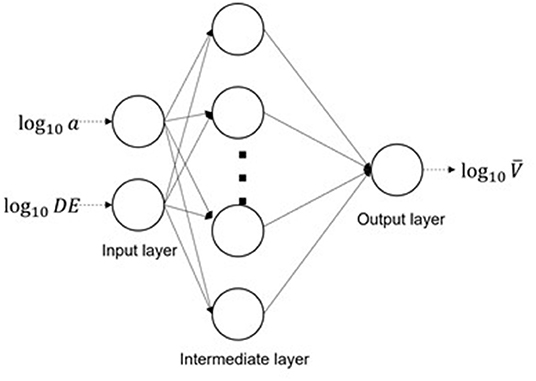Figure 2. Illustration of an artificial neural network. Each circle is a neuron, basic computation unit, in the network. Each arrow represents a computation connection from the neurons in last layer to the one in current layer.

For the lake volume prediction problem, xi is a 2-dimensional vector of surface area and lake elevation change in a log scale (Equation 8). xo is log10$\overline{\text{V}}$, the predicted volume (log scale), and y is the observed lake volume in log scale (log10V). Although the illustrated ANN architecture adopts a three-layer ANN, in practice, the depth of ANN and the number of neurons of intermediate layers can also vary and is determined empirically. For details of the ANN architecture we used, and other implementation details, please refer to Appendix A1.

Note that compared to statistical models, the parameters of ANN models are difficult to interpret mechanistically. Regression coefficients quantify the relationship between independent variables and target variables. In contrast, the learned parameters in ANN functionalize collectively without an explicit interpretation to understand the relationship between input features to outputs. Although some research has attempted to unveil its black-box mechanism (Montavon et al., 2018), its internal functions are still not as transparent and understandable as regression models and thus merits further research efforts to advance its progress.

### Temporal Variation of Total Lake Volume

Using the lake volume estimation model based on lake surface area alone, the temporal variation in the total volume of water in the region's lakes was determined using data from the Global Surface Water (GSW) observations program, which is based on LANDSAT imagery at a 30-meter resolution. The first regression formulation, Equation (1) was applied with surface areas derived from the digitized lake maps taken from the GSW data set for the period 2002 through 2015. An example of a digitized map image for two lakes for two dates (one in 2012 and one in 2015) is illustrated in Figure 3. The digital cells show the locations where the satellite sensed the presence of water. The blue colored cells show the presence of water in both 2012 and 2015, while the magenta colored cells show the presence of water in 2015, but not in 2012. The surface areas for each lake in the region was determined for each year (for the month of June), the areas were substituted into the regression model (Equation 1) to estimate the volume for each lake, and the total of water volume in the region was calculated by the sum of volumes for all lakes.

FIGURE 3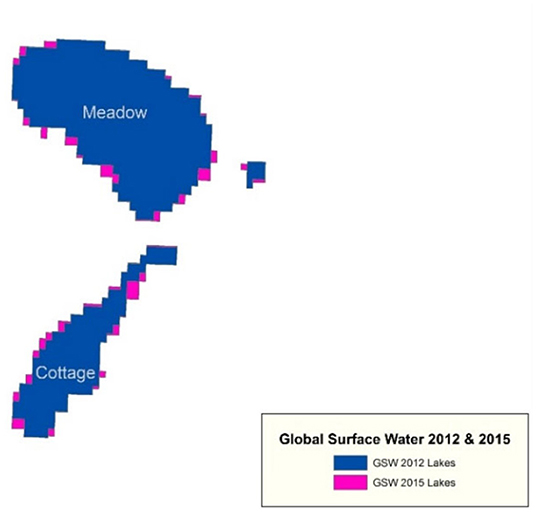Figure 3. The digital map for two lakes showing the presence of water in the cells; the magenta cells are locations where water was present in 2015 but not in 2012.

## Results

### Lake Surface Area Model

The bathymetric data for the 816 lakes were used to perform a regression by fitting to the measured surface area and the lake volume calculated from the bathymetric information. The model fit yielded

This model explained 83% of the variability of the lake volume. A plot of the predicted and observed lake volume using this regression is presented in Figure 4.

FIGURE 4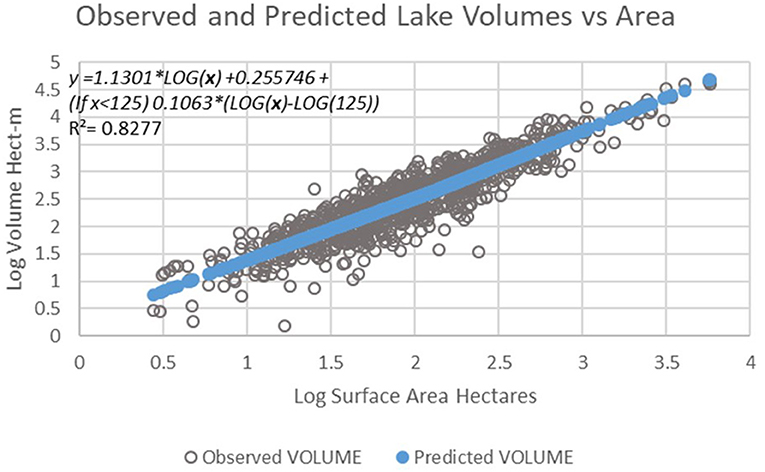Figure 4. Plot of predicted lake volume vs. observed lake volume for the 816 lakes using the simple regression on lake surface area model.

### Heathcote Method

#### All Lakes Pooled

Equation (2) represents the Heathcote et al. (2015) model for lake volume. The independent variables in this equation were determined as the best predictors based on the Pearson partial correlation coefficient and AIC test (Tables 2, 3). When comparing the known and predicted lake volumes, the model explained 82% of the variation in lake volume [R2 = 0.82, F(1,812) = 3,811, p < 2.2e−16] (Figure 5). The surface area and elevation change accounted for 82% and 2% of the variation within the model, respectively. The RSE for the model was 0.282 log10 m3. The coefficients for the all-lakes pooled data model were c = 1.17 and d = 0.07. The total lake volume predicted by the model for the pooled lakes was 7.5% different from the known total volume for the 816 lakes (Figure 5).

TABLE 2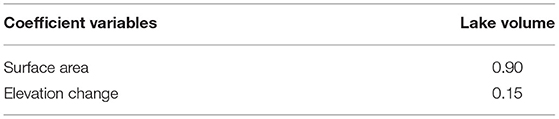Table 2. Pearson partial correlation coefficient tested to determine correlation strength of independent variables to lake volume.

TABLE 3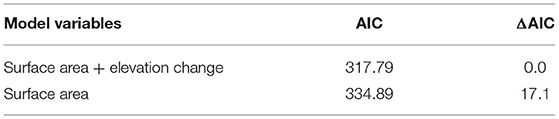Table 3. Akaike information criteria (AIC) and ΔAIC for the different predictive models tested for determining lake volume.

FIGURE 5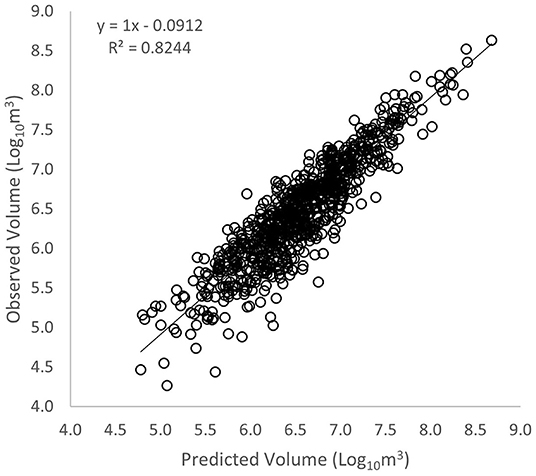Figure 5. Heathcote method of observed vs. predicted lake volumes for a linear regression model [R2 = 0.82, F(1,812) = 3,811, p < 2.2e−16].

#### Lakes Grouped by Size

Splitting the lake regression analysis by surface area resulted in an 83% explanation of the variation in lake volume [R2 = 0.83, F(1,812) = 3,835, p < 2.2e−16] (Table 4). The RSE for the model was 0.281 log10 m3. The coefficients c and d were different for each category of lake area, with c ranging from 0.78 to 1.26, and d ranging from −0.04 to 0.75. The total lake volume predicted by the size-segregated regression equations was 1.9% different from the known total lake volume (Table 4).

TABLE 4

#### Lakes Grouped by Watershed

Grouping the lakes by watershed resulted in the model explaining 84% of the variation in lake volume [R2 = 0.84, F(1,814) = 4,342, p < 2.2e−16] (Table 5). The RSE for the model was 0.269 log10 m3. The coefficients c and d were different for each category of watershed, with c ranging from 0.91 to 1.67, and d ranging from −0.30 to 0.78. The total lake volume predicted by the watershed-segregated regression equations was 2.6% different from the known total lake volume (Table 5).

TABLE 5

Using the different groupings of lakes, the total volumes were calculated for the 40,054 lakes within the region (Table 6). When comparing the three lake groupings, surface size grouping resulted in the highest lake volume with 1,236,436 hectare-meters while the model with all the lakes pooled yielded the lowest lake volume with 1,179,284 hectare-meters, a 4.7% difference (99% confidence interval 1,152,266–1,247,112 hectare-meters).

TABLE 6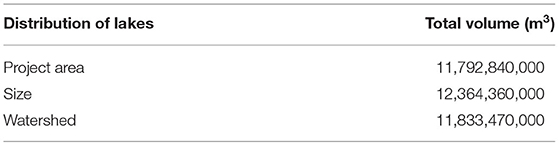Table 6. Comparison of total volume of the 40,054 lakes based on three approaches of Heathcote et al. (2015) method (Mille Lacs Lake not included).

### Cael Method

The Cael et al. (2017) method uses surface area which is the most significant variable to determine lake volume as seen in the Pearson partial correlation coefficient (Table 2). The analysis of Cael et al. was for lakes sampled from the US, Canada, and Sweden, and their analysis yielded a Hurst coefficient of 0.41. In our study of the 816 lakes the Cael et al. model yielded

For this model result, the Hurst coefficient is 0.34 which is within the theoretical range (0.4±0.1) for the earth's surface.

When comparing the known and predicted lake volumes based on Equation 11, the model explained 82% of the variation in volume for individual lake volumes [R2 = 0.82, F(1,812) = 3,697, p < 2.2e−16] (Figure 6). For this same regression equation, the total observed volume to the predicted volumes of the 816 lakes were compared. Our predictions were 1.4% different than that of the observed volume total (Table 7). The RSE for the model was 0.296 log10 m3. After calculating the total volume with the 40,054 lakes by both methods, the difference between the Heathcote et al. (2015) and the Cael et al. (2017) methods for all the lakes pooled was 3% (Table 8).

FIGURE 6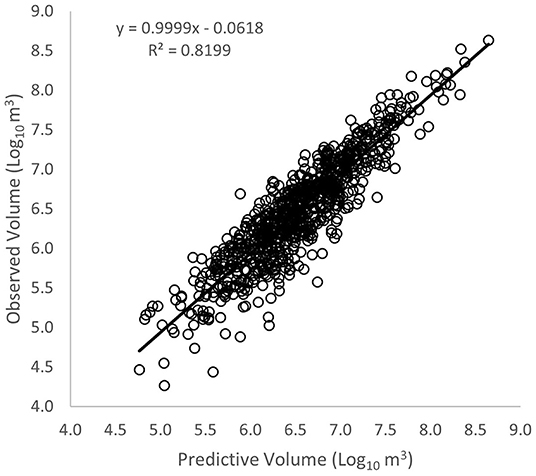Figure 6. Cael method of observed vs. predicted lake volumes for a linear regression model [R2 = 0.82, F(1,812) = 3,697, p < 2.2e−16].

TABLE 7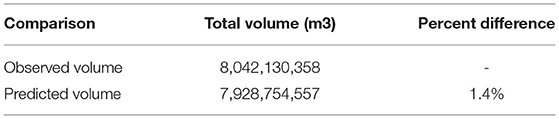Table 7. Using Cael et al. (2017) method, percent difference between the 816 observed lake volumes and the predicted volumes.

TABLE 8

### Machine Learning Method

All ML models were trained using the lake surface area and land surface elevation change, both of which are used in the Heathcote method while the Cael method uses only surface area. Therefore, we benchmarked ML methods against the Heathcote method. Without further grouping lake data based on the watershed location or lake surface area size, we used the full dataset for the purpose of investigating ML modeling ability in contrast to statistical regression models. To allow a fair comparison between machine learning methods and regression methods, the Heathcote method is evaluated using the cross-validation approach as well. Note that 5-fold cross-validation will evaluate the models using five different portions of the testing data and thus yield five different testing metrics. The less variant those testing metrics are, the more stable the corresponding models behave. As shown in Table 9, averages of the R2 and RMSE values across 5-fold validations are reported. Meanwhile, the standard deviation across 5-fold validations is also reported to show the stability of the model performance. Among all the ML models, although the ANN testing performance is less stable during cross-validation than the Heathcote method, ANN exhibits the best predictive performance with a RMSE of 0.286 and a R2 of 0.819 in contrast to the Heathcote method (0.296 RMSE and 0.811 R2). Besides, SVR (0.291 RMSE score and 0.819 R2) also achieves slightly better predictive performance than the Heathcote method. Both RF and GT yield a predictive performance slightly worse than, if not comparable to, the Heathcote method. The result is that the ANN model yielded the best predictive performance.

TABLE 9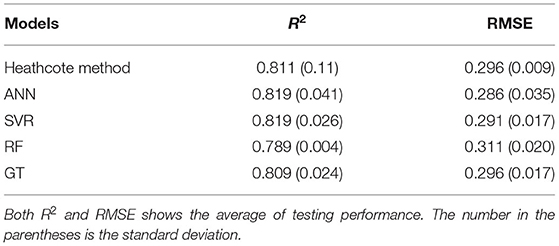Table 9. ML methods comparison against the Heathcote method results in a 5-fold cross validation test.

### Temporal Variation of Total Lake Volume in Central Minnesota Region

The data acquired from the GSW observation program was used to determine the surface areas of lakes on an annual basis for the study region. Those surface areas for the over 40,000 lakes were substituted into the regression model (Equation 1) and the volumes summed for all lakes. The resulting temporal variation of the total lake water stored (in equivalent mm) in the region is illustrated in Figure 7. There is a clear drop in water stored in the lakes in 2011–2012. Those years corresponded to a period of rainfall deficit.

FIGURE 7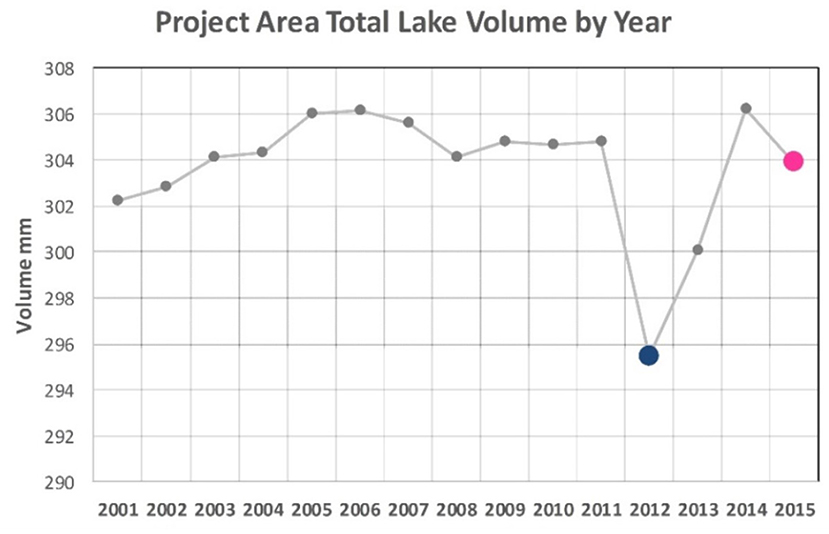Figure 7. The temporal variation in total water stored in the lakes of the region. Note the sharp drop in volume in the year 2011–2012, and the gradual recovery in following 2 years.

## Discussion

### Regression Methods

All three regression models, the simple regression given by Equation (1), the regression given by the Heathcote et al. (2015) model (Equation 2), and the Cael et al. (2017) model (Equation 3), provided fairly accurate predictions of the lake volumes for the 816 surveyed lakes. Among these, the Heathcote et al. model provided the best representation of the known individual lake volumes, while the Cael et al. model provided the best representation of the total volume of lake water in the region.

When comparing our research to the Heathcote et al. (2015) research, the lake surface area of lakes in Central Minnesota has a larger correlation to lake volume than that of the buffer elevation difference. This may be because of there being a smaller range of elevation within the study area, being a relatively flat region, resulting in the elevation difference in the buffer having a weaker relationship. The Heathcote et al. (2015) study compared 433 lakes selected from five different regions, two of which were situated in a mountainous region. When comparing the five regions, the mountainous region models produced the most accurate lake volumes as well as the highest R2 (R2 > 0.90). The regions with less elevation change such as the Eastmain region resulted in R2 similar to the results reported herein for Central Minnesota's R2 (R2 ≈ 0.80). This affirms the hypothesis that when the elevation has a larger range, the estimate of lake volume will have a stronger correlation to surface elevation change (Heathcote et al., 2015).

Among the three groupings of lakes using the Heathcote et al. (2015) procedure, determining lake volume by watershed resulted in the best prediction. A reason why the watershed grouping was the best prediction when compared to the known volumes is most likely that the lakes within a given subregion (or watershed) are almost all formed by the same geomorphic process, resulting in the lakes' formation being similar. Like Heathcote et al. (2015), we assumed that similar processes formed the lake and their landscape.

One issue with the analysis for all of the methods, regression and machine learning is that no bathymetric data exist for lakes smaller than 104 m2 surface area. To fill in this data, it was assumed that a lake smaller than 104 m2 surface area had an average depth of 0.5 m. This, of course, imposes an error in the data for a very large number of lakes that exist in the region. The predominance of larger lakes in the bathymetric data set is clear from Table 1, and it is clear from the estimates of total lake volume for the region that most of the total volume, about 66%, is contained in the 816 recorded lakes. The remaining 39,000+ lakes for which estimates were made contained the smaller fraction of the total volume. One improvement that could be made for the development of the prediction models would be to increase the amount of bathymetric data for the lakes in the small size range.

Another source of error in the analysis for the Heathcote et al. (2015) model was the use of a 1/3 arc-second DEM (U.S. Geological Survey, 2017). This approach essentially eliminated elevation data for lakes smaller than 104 m2 due to the lakes being too small for the DEM to pick up the elevation difference. For further research, DEM data with better resolution should be used in order to predict volumes more accurately by obtaining the buffer elevations from the smaller lakes.

It is not clear why the regression coefficient for the elevation change was negative for some of the data sets involving watershed groupings and lake area groupings. Theoretically, the coefficients should be positive. Perhaps the resulting negative coefficients occurred from less accurate elevation measurements resulting from the coarse DEM resolution. Further analysis is needed to determine the cause of the negative coefficients.

While this study is only limited to central Minnesota, an independent study covering the full state was completed to determine if the Heathcote et al. (2015) approach can accurately predict the lake volume for lakes across the entire state of Minnesota. For example, using the surface area and elevation change for lakes >4,047 m2 across the entire state of Minnesota, Griffin et al. (2018) and Finlay (2019) used the Heathcote et al. (2015) method to estimate lake volumes for the purpose of quantifying the regional variability of DOM pools in the water column of the region's lakes. Based on preliminary research, the model explained 82% of the variation in the lake volume with over 1,000 lakes of 4,047 m2 or larger. This research reaffirmed that using the lake's surface area and surrounding landscape can be used to accurately predict a lake's volume and can be used in diverse geographic areas with little morphologic and bathymetric data available.

The results for the Cael et al. (2017) model yielded a Hurst coefficient of 0.34 for the lakes in the Central Minnesota region. Cael et al. applied the method to four regions some of which had topographic features more like the Central Minnesota landscapes (Sweden, Wisconsin, some parts of Quebec), while others were more mountainous, for example the Adirondack region of New York. The resulting Hurst coefficients derived for these different regions reflected the topography of the individual regions. The Hurst coefficients derived by Cael et al. were 0.24 for the Wisconsin region, 0.32 for Sweden, 0.33 for Quebec (data included mountainous as well as more flat regions), and 0.48 for the Adirondack region. With all regions combined the derived Hurst coefficient was 0.40. This demonstrated that the Hurst coefficient picks up the topographic features through the relationship formed between lake surface area and lake volume.

In order to see whether, like the Heathcote et al. (2015) method, the Cael et al. (2017) method can have its lakes grouped by size and watershed, the lakes were grouped by the same categories. The significance of predicting total lake volumes when comparing the total volume of known lakes within the region was decreased when splitting into groups. Meaning that grouping the lakes by surface area size and watershed did not produce any significant results. Therefore, having a larger set of lakes when comprising the Cael et al. (2017) model improves the predictability of total lake volume. Even though the Cael et al. (2017) method was unable to significantly predict total lake volume when grouped by surface area and watershed, both the Heathcote et al. (2015) and the Cael et al. (2017) method were both able to significantly predict volumes when pooling all lakes together.

While both methods predict lake volume, the Cael et al. (2017) method, by design, is better suited to predict a group of lakes rather than individual lakes. The Heathcote et al. (2015) method is better at predicting volume and depth for individual lakes and therefore can be used when calculating individual lake processes. Consequently, one method may be more advantageous than the other depending on what future research questions are being asked.

### Machine Learning Method

Although the popularity of ML seemingly makes it a strong candidate approach for our lake volume predictions, a drastic improvement of the lake volume prediction accuracy is not observed in our case. Even though, among them, the ANN yields the best performance and suggests that its modeling ability to capture complex data patterns is more pronounced than the other three alternative models.

RF yields the relatively worst performance, which is likely caused by the low dimensions of input features (2-D data of lake surface area and elevation change) and its data hungry characteristics. Prediction tasks often benefit from the RF modeling because RF automatically finds uniform input feature subspace. However, given a 2-D input feature, the advantage of subspace searching is not leveraged. Further, a collection of 816 lakes is not a rich dataset for RF and would easily make RF overfit the training data and produce worse testing performance.

GT and SVR yielded comparable performance to the regression method. ANN exhibited the best performance among the selected ML methods and is slightly better than regression approaches. The reason for such a negligible performance improvement is possibly because the Heathcote method has achieved a performance satisfactory enough that the performance improvement room for ANN is too small. As shown in Figure 8, the correlation between log10(A) and log10(V) is as high as 0.90, which suggests a limited non-linear complexity between input data and lake volume. Such a limited non-linear data pattern constrained the ANN predictive performance improvement in contrast to the Heathcote method.

FIGURE 8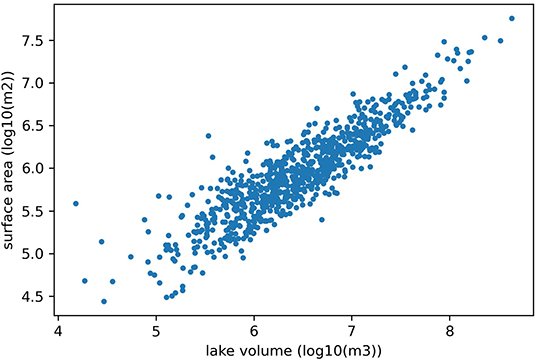Figure 8. The relationship between lake volume (log scale) and lake surface area (log scale).

All ML models show relatively more variant testing performance in contrast to the Heathcote method, which suggests the randomness in machine learning models and the uncertainty in its trainable parameters. On the contrary, the regression style Heathcote method preserves consistent testing performance (lower standard deviation of the testing performance in the cross-validation evaluation), which implies that linear regression models' generalization performance is more stable than ML for this problem.

Although ANN shows relatively better prediction accuracy, it does not have well-understood mechanisms underlying its explanatory power. For the Heathcote method, regression coefficients can offer sufficient interpretation to understand models. The positive regression coefficient of lake surface area and its statistical significance indicates the significant contribution of the surface area variable to lake volume estimation. However, this insight is missing for the ANN model.

Additionally, ANN only takes a 2-dimension input, which collectively groups all lakes together without any distinguishment among individual lakes. The model lacks distinct lake awareness information that might help more accurately predict volumes. It is likely that lake surface area and elevation change does not contain sufficient additional information for the volume prediction that is not already captured in the linear regression models. Therefore, it would be necessary to provide more physical information of lakes, such as, more lake geometry information, and surrounding land surface features, to further improve lake volume prediction accuracy.

Although the benefit of applying a machine learning model is not obvious for lake volume in our results, other bathymetric characterization of lakes, such as, lake depth may gain more from this approach. Heathcote et al. (2015) reported that a statistical model for predicting maximum lake depth only explains half of the system variance, which suggests that the majority of lake depth variance is difficult for statistical models to explain. Converse to the linear relationship between lake area and lake volume, relationships among other lake morphology features may be more complex. We hypothesize that this complexity is also accompanied with hidden non-linearities, which provides another research opportunity for implementing machine learning models and exploring their predictive capability in the future.

## Conclusion

We predicted lake volume through Central Minnesota using readily available morphologic data and a variety of previously published and novel methods. Three regression-based analysis methods and four machine learning methods were applied to develop predictions of lake volumes for over 40,000 lakes located in the central section of Minnesota. The methods were developed using detailed lake bathymetric data for 816 lakes located in the same region. The resulting prediction methods estimated the total volume of lake water in the region to be in the range of about 12 ± 0.2 km3.

The regression models included a regression on lake surface area, a model based on the Heathcote et al. (2015) model that included lake surface area and mean elevation change in a designated buffer area outside the lake area, and a model based on the Cael et al. (2017) model that utilized the theory of self-affine surfaces. Among the machine learning models, the ANN performed the best, and it was found that the ANN performance was slightly better than any of the regression models. The small incremental benefit in performance of the ANN method over the regression models is explained by the fact that the relation between log-transformed lake surface area and log-transformed lake volume is nearly linear. If the relation were more non-linear, the ML methods might have been able to provide a larger increase in performance. This is the power of ML approaches, in that they facilitate the development of data-driven models when the relations between variables are complex and non-linear. One immediate future need is to evaluate the ability of ML methods for prediction of lake maximum depth.

## Data Availability Statement

Publicly available datasets were analyzed in this study. This data can be found here: Lake data: https://hdl.handle.net/11299/211726, Global surface water: https://global-surface-water.appspot.com/download.

## Author Contributions

JN conceived the project idea and acquired the funding. CD conducted statistical method prediction and prepared the first draft of this manuscript with the guidance kindly offered from AH. XL conducted the ML experiments and wrote their counterparts in the manuscript. KH developed the volume-surface area regression model and analyzed the annual trend analysis. BW supervised the statistical analysis design. CD, XL, AH, and JN all proofread and revised the manuscript. All authors contributed to the article and approved the submitted version.

## Funding

This study was financially supported by the Legislative-Citizen Commission on Minnesota Resources (M.L. 2017, Chp. 96, Sec. 2, Subd. 04h).

## Conflict of Interest

The authors declare that the research was conducted in the absence of any commercial or financial relationships that could be construed as a potential conflict of interest.

## Publisher's Note

All claims expressed in this article are solely those of the authors and do not necessarily represent those of their affiliated organizations, or those of the publisher, the editors and the reviewers. Any product that may be evaluated in this article, or claim that may be made by its manufacturer, is not guaranteed or endorsed by the publisher.

## Acknowledgments

JN effort on this project was partially supported by the USDA National Institute of Food and Agriculture, Hatch/Multistate Project MN 12-109. BW effort on this project was partially supported by the USDA National Institute of Food and Agriculture, Hatch/Multistate Project MN 12-069.

## References

Adadi, A. (2021). A survey on data-efficient algorithms in big data era. J. Big Data. 8, 1–54. doi: 10.1186/s40537-021-00419-9

Akaike, H. (1974). A new look at the statistical model identification. IEEE Trans. Automat. Contr. 19, 716–723. doi: 10.1109/TAC.1974.1100705

Avand, M., Kuriqi, A., Khazaei, M., and Ghorbanzadeh, O. (2022). DEM resolution effects on machine learning performance for flood probability mapping. J. Hydro-Environ. Res. 40, 1–16. doi: 10.1016/j.jher.2021.10.002

Borchers, H. W. (2019). Package “pracma” (2.2.5). R Foundation for Statistical Computing, Vienna, Austria. Available online at: http://CRAN.Rproject.org/package=pracma

Breiman, L. (2001). Random forests. Mach. Learn. 45, 5–32. doi: 10.1023/A:1010933404324

Cael, B. B., Heathcote, A. J., and Seekell, D. A. (2017). The volume and mean depth of Earth's lakes. Geophys. Res. Lett. 44, 209–218. doi: 10.1002/2016GL071378

Chen, T., and Guestrin, C. (2016). XGBoost: a scalable tree boosting system. Knowled. Discov. Data Mining 16, 651–662. doi: 10.1145/2939672.2939785

Cortes, C., and Vapnik, V. (1995). Support-vector networks. Mach. Learn. 20, 273–297. doi: 10.1007/BF00994018

Crétaux, J. F., Abarca-del-Río, R., Bergé-Nguyen, M., Arsen, A., Drolon, V., Clos, G., et al. (2016). Lake volume monitoring from space. Surv. Geophys. 37, 269–305. doi: 10.1007/s10712-016-9362-6

Delaney, C. O. (2019). Estimating lake water volume using scale analysis (M.S.Thesis). University of Minnesota, St Paul, MN, United States.

Deoli, V., Kumar, D., Kumar, M., Kuriqi, A., and Elbeltagi, A. (2021). Water spread mapping of multiple lakes using remote sensing and satellite data. Arab. J. Geosci. 14, 1–15. doi: 10.1007/s12517-021-08597-9

Department of Natural Resources Division of Fish and Wildlife (2014). DNR Hydrography - Lakes and Open Water. Available online at: http://www.mngeo.state.mn.us/committee/standards/mgmg/metadata.htm%0A (accessed November 29, 2018).

Dreyfus, S. E. (1990). Artificial neural networks, back propagation, and the Kelley-Bryson gradient procedure. J. Guid. Control Dyn. 13, 926–928. doi: 10.2514/3.25422

Ferguson, R. I. (1986). River loads underestimated by rating curves. Water Resour. Res. 22, 74–76. doi: 10.1029/WR022i001p00074

Finlay, J. (2019). Assessment of surface water quality with satellite sensors. Final Report, project funded by the Legislative-Citizens Committee on Minnesota Resources, Legal Citation: M.L. 2016, Chp. 186, Sec. 2, Subd. 04i.

Griffin, C. G., Holmberg, K., Delaney, C., Olmanson, L. G., Brezonik, P. L., Nieber, J., et al. (2018). “Remote sensing of dissolved organic matter pools in lakes at regional scales,” in IGU Fall Meeting Conference, Vol. 2018 (Washington, DC: American Geophysical Union).

Håkanson, L., and Karlsson, B. (1984). On the relationship between regional geomorphology and lake morphometry-A Swedish example. Geografiska Annaler: Ser. A, Phys. Geograph. 66, 103–119. doi: 10.1080/04353676.1984.11880102

Håkanson, L., and Peters, R. H. (1995). Predictive Limnology. Amsterdam: SPB Academic.

Heathcote, A. J., del Giorgio, P. A., and Prairie, Y. T. (2015). Predicting bathymetric features of lakes from the topography of their surrounding landscape. Can. J. Fish. Aquat. Sci. 72, 643–650. doi: 10.1139/cjfas-2014-0392

Hirschberg, J., and Manning, C. D. (2015). language processing. Science. 349, 261–266. doi: 10.1126/science.aaa8685

Hollister, J. W., Milstead, W. B., and Urrutia, M. A. (2011). Predicting maximum lake depth from surrounding topography. PLoS ONE 6, e25764. doi: 10.1371/journal.pone.0025764

Jia, X., Willard, J., Karpatne, A., Read, J. S., Zwart, J. A., Steinbach, M., et al. (2020). Physics-guided machine learning for scientific discovery: an application in simulating lake temperature profiles. ACM IMS Trans. Data Sci. 2, 1–25. doi: 10.1145/3447814

Kim, S. (2015). Package “ppcor” (1.1). R Foundation for Statistical Computing, Vienna, Austria. Available online at: https://cran.r-project.org/web/packages/ppcor/ppcor.pdf

Kratzert, F., Klotz, D., Brenner, C., Schulz, K., and Herrnegger, M. (2018). Rainfall – runoff modelling using Long Short-Term Memory (LSTM) networks. Hydrol. Earth Syst. Sci. 22, 6005–6022. doi: 10.5194/hess-22-6005-2018

Kratzert, F., Klotz, D., Shalev, G., Klambauer, G., Hochreiter, S., and Nearing, G. (2019). Towards learning universal, regional, and local hydrological behaviors via machine learning applied to large-sample datasets. Hydrol. Earth Syst. Sci. 23, 5089–5110. doi: 10.5194/hess-23-5089-2019

LeCun, Y., Bengio, Y., and Hinton, G. (2015). Deep learning. Nature 521, 436–444. doi: 10.1038/nature14539

Leschinski, C. H. (2019). Package ‘MonteCarlo' (1.0.6). R Foundation for Statistical Computing, Vienna, Austria. Available online at: https://CRAN.R-project.org/package=MonteCarlo

McDonald, C. P., Rover, J. A., Stets, E. G., and Striegl, R. G. (2012). The regional abundance and size distribution of lakes and reservoirs in the United States and implications for estimates of global lake extent. Limnol. Oceanogr. 57, 597–606. doi: 10.4319/lo.2012.57.2.0597

Messager, M. L., Lehner, B., Grill, G., Nedeva, I., and Schmitt, O. (2016). Estimating the volume and age of water stored in global lakes using a geo-statistical approach. Nat. Commun. 7, 1–11. doi: 10.1038/ncomms13603

Minnesota Department of Natural Resources (2017). Lake Basin Morphology. St Paul, MN: Minnesota DNR, Division of Fish and Wildlife.

Montavon, G., Samek, W., and Müller, K. R. (2018). Methods for interpreting and understanding deep neural networks. Digit. Signal Process. A Rev. J. 73, 1–15. doi: 10.1016/j.dsp.2017.10.011

Obertegger, U., Flaim, G., Braioni, M. G., Sommaruga, R., Corradini, F., and Borsato, A. (2007). Water residence time as a driving force of zooplankton structure and succession. Aquat. Sci. 69, 575–583. doi: 10.1007/s00027-007-0924-z

Pekel, J.-F., Cottam, A., Gorelick, N., and Belward, A. S. (2016). High-resolution mapping of global surface water and its long-term changes. Nature 540, 418–422. doi: 10.1038/nature20584

Renard, F., Candela, T., and Bouchaud, E. (2013). Constant dimensionality of fault roughness from the scale of micro-fractures to the scale of continents. Geophys. Res. Lett. 40, 83–87. doi: 10.1029/2012GL054143

RStudio Team (2016). RStudio: Integrated Development for R. Boston, MA: RStudio, Inc. (1.1.456).

Sejnowski, T. J. (2020). The unreasonable effectiveness of deep learning in artificial intelligence. Proc. Natl. Acad. Sci. U. S. A. 117, 30033–30038. doi: 10.1073/pnas.1907373117

Shen, C., Laloy, E., Elshorbagy, A., Albert, A., Bales, J., Chang, F. J., et al. (2018). HESS opinions: incubating deep-learning-powered hydrologic science advances as a community. Hydrol. Earth Syst. Sci. 22, 5639–5656. doi: 10.5194/hess-22-5639-2018

Shukla, R., Kumar, P., Vishwakarma, D. K., Ali, R., Kumar, R., and Kuriqi, A. (2022). Modeling of stage-discharge using back propagation ANN-, ANFIS-, and WANN-based computing techniques. Theor. Appl. Climatol. 147, 867–889. doi: 10.1007/s00704-021-03863-y

Sobek, S., Nisell, J., and Folster, J. (2011). Predicting the volume and depth of lakes from map-derived parameters. Inland Waters 1, 177–184. doi: 10.5268/IW-1.3.426

U.S. Geological Survey. (2017). 1/3rd Arc-Second Digital Elevation Models (DEMs) – USGS National Map 3DEP Downloadable Data Collection. Reston, VA: U.S. Geological Survey.

## Appendix A1

Hyper-parameters of the applied ML models are listed below. Those values were determined after hyper-parameter tuning.

### Artificial Neural Network

Activation function for each layer: ReLu.

Model architecture: input (2d) -> 4d -> 16d -> 32d -> (output) 1d Optimization algorithm: Adam optimizer (learning rate: 0.001).

### Random Forest

Number of trees: 100

Maximum tree depth: 8.

### Support Vector Machine

Number of trees: 80.

## Appendix A2

### Abbreviation Glossary

MnDNR, Minnesota Department of Natural Resources.

HUC-8, Hydrologic unit codes.

GSW, Global surface water.

DEM, Digital Elevation map.

AIC, Akaike information criteria.

RMSE, Root-mean-square error.

RSE, Relative standard error.

ML, Machine Learning.

ANN, Artificial neural network.

SVR, Support vector regression.

RF, Random forests.

CDOM, colored dissolved organic matter.

DOC, dissolved organic carbon.

LANDSAT, Satellite that studies and photographs the surface by using remote-sensing techniques.

### Variable Glossary

V, Volume.

a, b, c, d, empirical constants.

A, Lake surface area.

D, Buffer distance from the shoreline outward.

DE25, 25% of the average elevation changes within the buffer.

H, Hurst Coefficient.

ζ, volume-area scaling exponent.

κ, proportionality coefficient.

ε, error term.

xi, input data.

xo, output target variable.

xm, intermediate layer output.

σ, non-linear operator.

, trainable parameters (weight matrix in neural network layers).

bm, bo, trainable parameters (bias terms in neural network layers).

L, Loss Function.

N, Number of input data records.

y, observed data.

Keywords: bathymetry, lake volume, scale analysis, machine learning, Minnesota

Citation: Delaney C, Li X, Holmberg K, Wilson B, Heathcote A and Nieber J (2022) Estimating Lake Water Volume With Regression and Machine Learning Methods. Front. Water 4:886964. doi: 10.3389/frwa.2022.886964

Received: 01 March 2022; Accepted: 06 April 2022;
Published: 16 June 2022.

Edited by:

Tongren Xu, Beijing Normal University, China

Reviewed by:

Georgia A. Papacharalampous, Czech University of Life Sciences, Czechia
Alban Kuriqi, Universidade de Lisboa, Portugal

Copyright © 2022 Delaney, Li, Holmberg, Wilson, Heathcote and Nieber. This is an open-access article distributed under the terms of the Creative Commons Attribution License (CC BY). The use, distribution or reproduction in other forums is permitted, provided the original author(s) and the copyright owner(s) are credited and that the original publication in this journal is cited, in accordance with accepted academic practice. No use, distribution or reproduction is permitted which does not comply with these terms.

*Correspondence: John Nieber, nieber@umn.edu